# 实验项目：

1、利用复化梯形公式计算定积分
2、计算Ackerman函数
3、编写计算x的y次幂的递归函数getpower(int x,int y)，并在主程序中实现输入输出
4、编写计算学生年龄的递归函数
5、编写递归函数实现Ackman函数

# 姓名：宋梦南  实验地点：教学楼514  实验时间：2019.5.16

## 一、实验目的与要求

### 1、利用复化梯形公式计算定积分

• 掌握C语言中定义函数的方法
• 掌握通过“值传递”调用函数的方法

### 2、计算Ackerman函数

• 掌握递归函数的设计方法
• 进一步练习阅读检查与调试修改C程序的方法

### 3、编写计算x的y次幂的递归函数getpower(int x,int y)，并在主程序中实现输入输出

• 写出解决该问题的递归算法：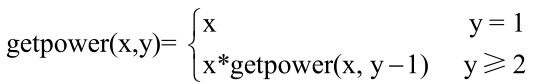• 在递归函数中，使用数字1 作为回归条件；
• 在递归函数中，使用 if_else 语句根据条件的真假来决定是递推还是回归。

### 4、编写计算学生年龄的递归函数

• 写出解决该问题的递归算法：
递归公式如下，根据公式容易写出递归程序。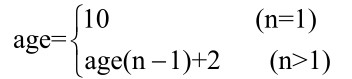• 在递归函数中，使用数字1 作为回归条件；
• 在递归函数中，使用if_else语句根据条件的真假来决定是递推还是回归。

### 5、编写递归函数实现Ackman函数

• 根据递归公式编写递归函数；
• 在递归函数中，使用if_else语句根据条件的真假来决定是递推还是回归。

## 1.实验练习：6.4.2.2

### 1.问题描述：

（1）编制一个函数sab（a,b,n）,其功能为利用复化梯形公式计算定积分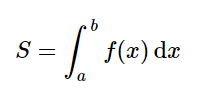（2）编制一个主函数以及计算被积函数值的函数f(x)，在主函数中调用（1）中的函数sab(a,b,n)计算并输出下列积分值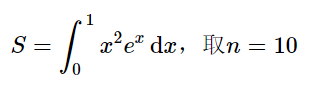3）编制另一个主函数以及计算被积函数值的函数f(x)，在主函数中调用（1）中的函数sab(a,b,n)计算并输出下列积分值。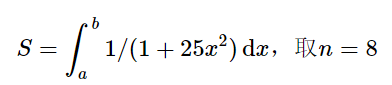（4）要求画出模块sab()的流程图。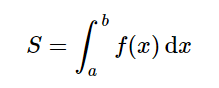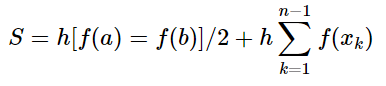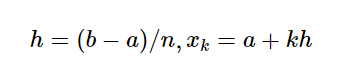### 2.流程图：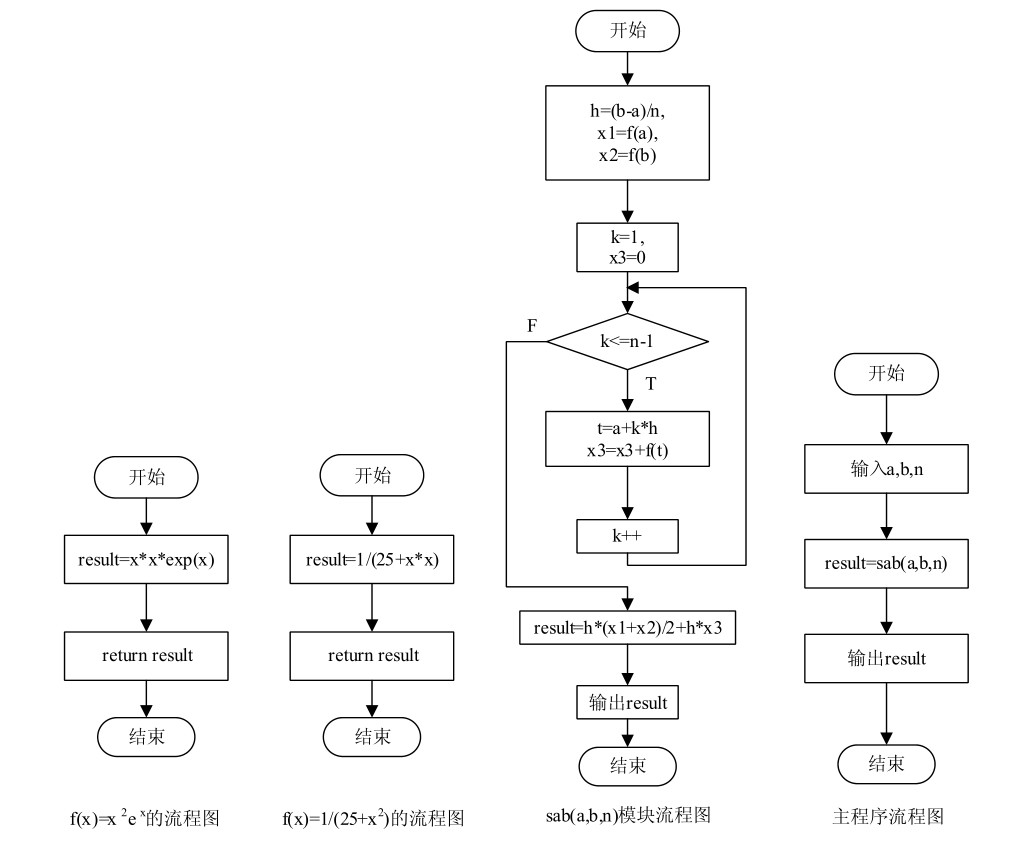### 3.实验代码：

```#include<stdio.h>
double f(double x);
double sab(double a, double b,int n)
{
int k;
double h,result,x1,x2,x3=0,t;
h=(b-a)/n;
x1=f(a);
x2=f(b);
for (k=1;k<=n-1;k++)
{
t=a+k*h;
x3=x3+f(t);
}
result=h*(x1+x2)/2+h*x3;
return result;
}```
```#include<stdio.h>
#include<math.h>
#include"sab.h"
double f(double x)
{
double result;
result=x*x*exp(x);
return result;
}
main()
{
double a,b,result;
int n;
printf("please input double a,b and integer n:");
scanf("%lf,%lf,%d",&a,&b,&n);
result=sab(a,b,n);
printf("sab(%lf,%lf,%d)=%lf",a,b,n,result);
return 0;
}```
```#include<stdio.h>
#include"sab.h"
double f(double x)
{
double result;
result=1/(25+x*x);
return result;
}
main()
{
double a,b,result;
int n;
printf("please input double a,b and integer n:");
scanf("%lf,%lf,%d",&a,&b,&n);
result=sab(a,b,n);
printf("sab(%lf,%lf,%d)=%lf",a,b,n,result);
return 0;
}```

### 5.运行效果：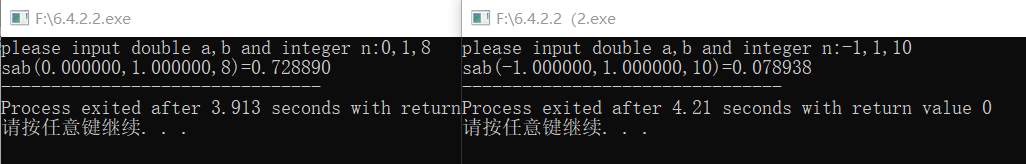## 2.实验练习：6.4.2.3

### 1.问题描述：

(1)根据方法说明，编制计算Ackerman函数的递归函数ack(n,x,y)。
(2)编制一个主函数，由键盘输入n,x,y,调用(1)中的函数ack(n,x,y)，计算Ackerman函数。
(3)在主函数中，输入之前要有提示，并检查输入数据的合理性，若输入的数据不合理，则输出错误信息，输出要有文字说明。
(4)输入(n,x,y)=(2,3,1)运行该程序。然后自定义几组数据再运行该程序。

Ackerman函数的定义如下：

n,x,y为非负整数，且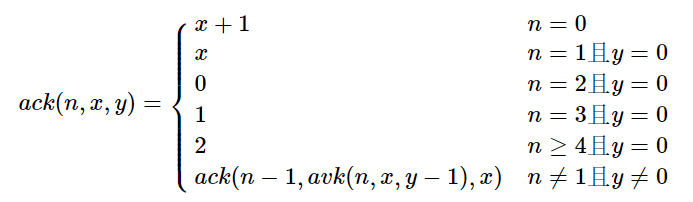### 2.流程图：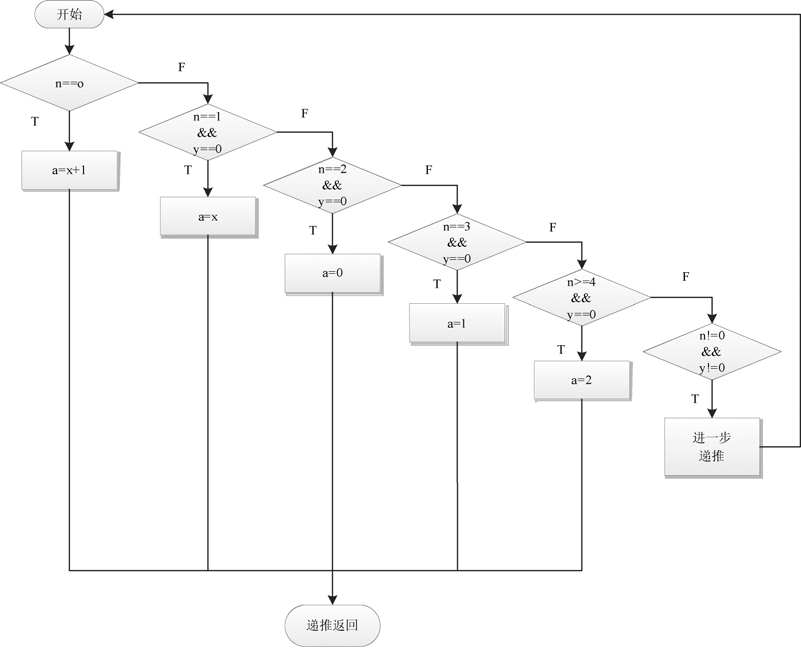### 3.实验代码：

```#include<stdio.h>
int Ack(int n,int x,int y)
{
int a;
if(n==0)
a=x+1;
else if(n==1&&y==0)
a=x;
else if(n==2&&y==0)
a=0;
else if(n==3&&y==0)
a=1;
else if(n>=4&&y==0)
a=2;
else if(n!=0&&y!=0)
a=Ack(n-1,Ack(n,x,y-1),x);
return a;
}
main()
{
int n,x,y,result;
printf("please input n,x,y:");
scanf("%d,%d,%d",&n,&x,&y);
if(n&&x&&y<0)
printf("error!");
else
result=Ack(n,x,y);
printf("Ack(%d,%d,%d)=%d\n",n,x,y,result);

}```

### 5.运行效果：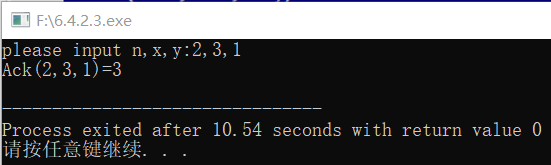## 3.实验练习：6.4.3.1

### 2.流程图：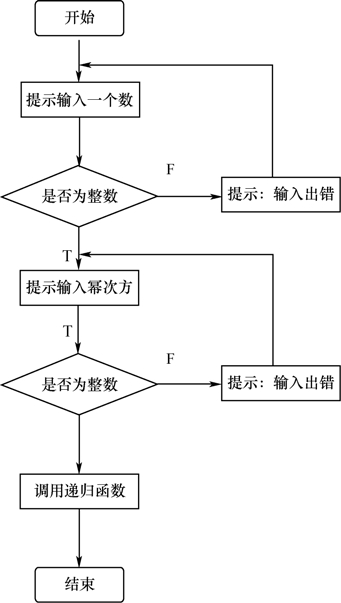### 3.实验代码：

```#include<stdio.h>
long getpower(int x,int y)
{
if(y==1)
return x;
else if(y>=2)
return x*getpower(x,y-1);
}
main()
{
int num,power;
long answer;
printf("输入一个数：");
scanf("%d",&num);
printf("\n输入幂次方:");
scanf("%d",&power);
answer=getpower(num,power);
printf("\n结果是：%ld",answer);

}```

### 5.运行效果：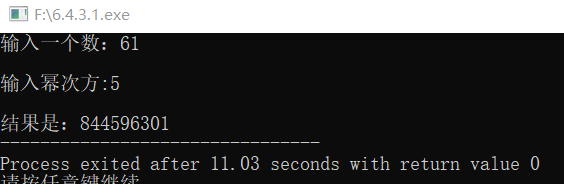## 4.实验练习：6.4.3.2

### 2.流程图：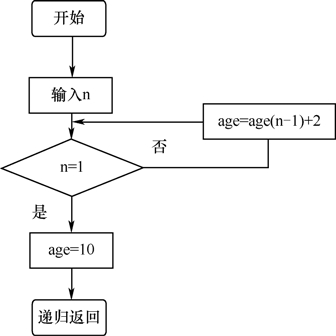### 3.实验代码：

```#include<stdio.h>
int age(int n)
{
int c;
if(n==1)
c=10;
else
c=age(n-1)+2;
return c;
}
main()
{
int a,n=5;
a=age(n);
printf("第%d位同学的年龄为%d",n,a);
}```

### 5.运行效果：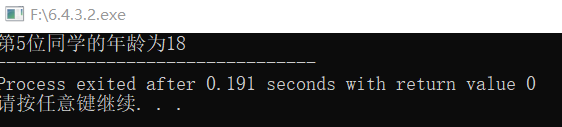## 5.实验练习：6.4.3.3

### 1.问题描述：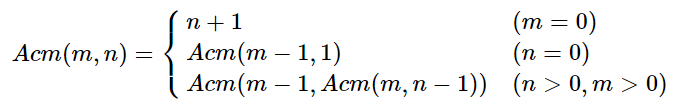### 2.流程图：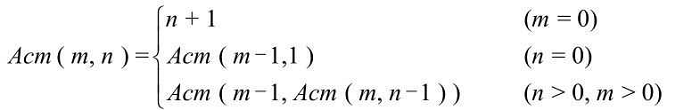### 3.实验代码：

```#include<stdio.h>
Acm(int m,int n)
{
int a;
if (m==0)
a=n+1;
else if (n==0)
a=Acm(m-1,1);
else if (n>0&&m>0)
a=Acm(m-1,Acm(m,n-1));
return a;
}
main()
{
int b,c;
b=Acm(2,1);
c=Acm(3,2);
printf("Acm(2,1)=%d\nAcm(3,2)=%d",b,c);
return 0;
}```

### 5.运行效果：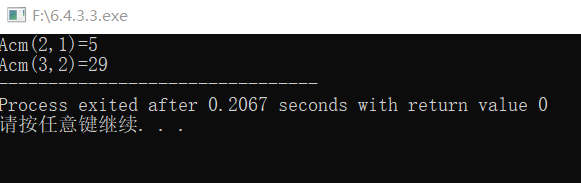## 三、实验小结

最主要的还是要知道递归函数的定义，才能根据函数写出代码，通常情况下，都是要用if语句分情况讨论，在写的时候注意不要落下某一种情况，所以写完要记得检查一遍，当代码没有错误时，但是却运行不出来的时候，就要看看是不是某个数的定义类型有误，或者是标点符号漏错的问题。在写递归函数的时候，有时候几个程序都要调用一个函数，那么就可以把它独立的放到一个文件中，会更方便。

0条评论